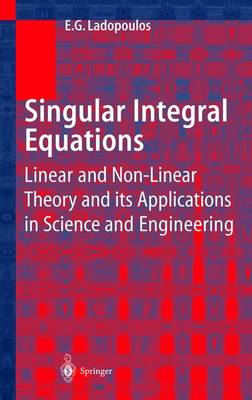•Singular Integral Equations: Linear and Non-linear Theory and its Applications in Science and Engineering (Hardback)

(author)
£249.99
Hardback 552 Pages / Published: 06/06/2000
• We can order this

Usually dispatched within 3 weeks

The present book deals with the finite-part singular integral equations, the multidimensional singular integral equations and the non-linear singular integral equations, which are currently used in many fields of engineering mechanics with applied character, like elasticity, plasticity, thermoelastoplasticity, viscoelasticity, viscoplasticity, fracture mechanics, structural analysis, fluid mechanics, aerodynamics and elastodynamics. These types of singular integral equations form the latest high technology on the solution of very important problems of solid and fluid mechanics and therefore special attention should be given by the reader of the present book, who is interested for the new technology of the twentieth-one century. Chapter 1 is devoted with a historical report and an extended outline of References, for the finite-part singular integral equations, the multidimensional singular integral equations and the non-linear singular integral equations. Chapter 2 provides a finite-part singular integral representation analysis in Lp spaces and in general Hilbert spaces. In the same Chapter are investigated all possible approximation methods for the numerical evaluation of the finite-part singular integral equations, as closed form solutions for the above type of integral equations are available only in simple cases. Also, Chapter 2 provides further a generalization of the well known Sokhotski-Plemelj formulae and the Nother theorems, for the case of a finite-part singular integral equation.

Publisher: Springer-Verlag Berlin and Heidelberg GmbH & Co. KG
ISBN: 9783540672302
Number of pages: 552
Weight: 2180 g
Dimensions: 235 x 155 x 31 mm
Edition: 2000 ed.